Test: Ratio And Proportion, Indices, Logarithms - 1

# Test: Ratio And Proportion, Indices, Logarithms - 1

Test Description

## 40 Questions MCQ Test Business Mathematics and Logical Reasoning & Statistics | Test: Ratio And Proportion, Indices, Logarithms - 1

Test: Ratio And Proportion, Indices, Logarithms - 1 for CA Foundation 2023 is part of Business Mathematics and Logical Reasoning & Statistics preparation. The Test: Ratio And Proportion, Indices, Logarithms - 1 questions and answers have been prepared according to the CA Foundation exam syllabus.The Test: Ratio And Proportion, Indices, Logarithms - 1 MCQs are made for CA Foundation 2023 Exam. Find important definitions, questions, notes, meanings, examples, exercises, MCQs and online tests for Test: Ratio And Proportion, Indices, Logarithms - 1 below.
Solutions of Test: Ratio And Proportion, Indices, Logarithms - 1 questions in English are available as part of our Business Mathematics and Logical Reasoning & Statistics for CA Foundation & Test: Ratio And Proportion, Indices, Logarithms - 1 solutions in Hindi for Business Mathematics and Logical Reasoning & Statistics course. Download more important topics, notes, lectures and mock test series for CA Foundation Exam by signing up for free. Attempt Test: Ratio And Proportion, Indices, Logarithms - 1 | 40 questions in 40 minutes | Mock test for CA Foundation preparation | Free important questions MCQ to study Business Mathematics and Logical Reasoning & Statistics for CA Foundation Exam | Download free PDF with solutions
 1 Crore+ students have signed up on EduRev. Have you?
Test: Ratio And Proportion, Indices, Logarithms - 1 - Question 1

### Choose the most appropriate option. The inverse ratio of 11 : 15 is

Detailed Solution for Test: Ratio And Proportion, Indices, Logarithms - 1 - Question 1

The inverse ratio of 11:15 is 15:11

Test: Ratio And Proportion, Indices, Logarithms - 1 - Question 2

### Choose the most appropriate option. The ratio of two quantities is 3 : 4. If the antecedent is 15, the consequent is

Detailed Solution for Test: Ratio And Proportion, Indices, Logarithms - 1 - Question 2

Let the numbers be 3x , 4x
Given ; the antecedent is 15

⇒ 3x = 15
⇒  x = 5

Therefore; 4x = 4*5 = 20

The consequent is 20.

Test: Ratio And Proportion, Indices, Logarithms - 1 - Question 3

### The ratio of the quantities is 5 : 7. If the consequent of its inverse ratio is 5, the antecedent is

Detailed Solution for Test: Ratio And Proportion, Indices, Logarithms - 1 - Question 3

The ratio of two quantities is 5:7
The inverse ratio is 7:5
The antecedent is 7

Test: Ratio And Proportion, Indices, Logarithms - 1 - Question 4

The ratio compounded of 2 : 3, 9 : 4, 5 : 6 and 8 : 10 is

Detailed Solution for Test: Ratio And Proportion, Indices, Logarithms - 1 - Question 4

2×3×9×4×5×6×8×10 = 720:720
which gives 1:1

Test: Ratio And Proportion, Indices, Logarithms - 1 - Question 5

The duplicate ratio of 3 : 4 is

Detailed Solution for Test: Ratio And Proportion, Indices, Logarithms - 1 - Question 5

Given ratio is 3:4

since duplicate ratio is by a^2 :b^2

then we have 9:16

Test: Ratio And Proportion, Indices, Logarithms - 1 - Question 6

The sub duplicate ratio of 25 : 36 is

Detailed Solution for Test: Ratio And Proportion, Indices, Logarithms - 1 - Question 6

Given is 25: 36
The sub- duplicate ratio is given by √a :√b
Hence we have √25 : √36 is 5 : 6

Test: Ratio And Proportion, Indices, Logarithms - 1 - Question 7

The ratio compounded of 4:9, and the duplicate ratio of 3:4,the triplicate ratio of 2:3 and 9:7 is

Detailed Solution for Test: Ratio And Proportion, Indices, Logarithms - 1 - Question 7

The duplicate ratio of 3:4 is 32/42 = 9/16.
The triplicate ratio of 2:3 is 23/33 = 8/27.

Now we have 4 ratios: 4:9, 9:16, 8:27 and 9:7.

We have to calculate the ratio compounded of all these ratios.

That will be (4 x 9 x 8 x 9)/(9 x 16 x 27 x 7) = 2/21.

Test: Ratio And Proportion, Indices, Logarithms - 1 - Question 8

The sub triplicate ratio of 8 : 27 is

Detailed Solution for Test: Ratio And Proportion, Indices, Logarithms - 1 - Question 8

Sub triplicate ratio of 8:27 is 3√8:3√27=2:3.

Test: Ratio And Proportion, Indices, Logarithms - 1 - Question 9

The ratio compounded of 4 : 9 and the duplicate ratio of 3 : 4 is

Detailed Solution for Test: Ratio And Proportion, Indices, Logarithms - 1 - Question 9

Dupliate ratio of 3:4, will be the square of the ratio
So, this will be 9:16
And the compounded ratio will be product of two ratios:
4:9 x 9:16 = 1:4 ( 9 will be cancelled and 16 by 4 )

Test: Ratio And Proportion, Indices, Logarithms - 1 - Question 10

If log (2a - 3b) = log a - log b, then a =

Detailed Solution for Test: Ratio And Proportion, Indices, Logarithms - 1 - Question 10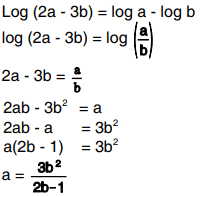Test: Ratio And Proportion, Indices, Logarithms - 1 - Question 11

The ratio compounded of duplicate ratio of 4 : 5, triplicate ratio of 1 : 3, sub duplicate ratio of 81 : 256 and sub triplicate ratio of 125 : 512 is

Detailed Solution for Test: Ratio And Proportion, Indices, Logarithms - 1 - Question 11

The duplicate ratio of 4 : 5 is 4^2 : 5^2 i.e. 16 : 25

The triplicate ratio of 1 : 3 is 1^3 : 3^3 = 1 : 27

Sub duplicate ratio of 81 : 256 is (81)^1/2 : (256)^1/2 i.e. 9 : 16

Sub triplicate ratio of 125 : 512 is (125)^1/3: (512)^1/3 i.e. 5 : 8

Therefore the compounded ratio of (16:25) ; (1:27); (9:16) and (5:8) is:

16/25 x 1/27 x 9/16 x 5/8 = 9 x 5/25 x 27 x 8 = 1/(5 x 3 x 8) = 1/120.

Test: Ratio And Proportion, Indices, Logarithms - 1 - Question 12

If a : b = 3 : 4, the value of (2a+3b) : (3a+4b) is

Detailed Solution for Test: Ratio And Proportion, Indices, Logarithms - 1 - Question 12

A/b=3/4
So,
[(2 x 3)+(3 x 4)]/[(3 x 3)+(4 x 4)]
(6+12)/(9+16)
18/25

Test: Ratio And Proportion, Indices, Logarithms - 1 - Question 13

Two numbers are in the ratio 2 : 3. If 4 be subtracted from each, they are in the ratio 3 : 5.The numbers are

Detailed Solution for Test: Ratio And Proportion, Indices, Logarithms - 1 - Question 13

Let no. 2x and 3x then
(2x-4) / (3x-4) = 3 / 5
10x-20 = 9x-12
x=8
Now, numbers are: 2×8 = 16 & 3×8 = 24

Test: Ratio And Proportion, Indices, Logarithms - 1 - Question 14

The angles of a triangle are in ratio 2:7:11. The angles are

Detailed Solution for Test: Ratio And Proportion, Indices, Logarithms - 1 - Question 14

Sum of angle of a triangle is 180 so,
2x + 7x + 11x = 180
20x = 180
x = 9

now put the value of x than we will get the angles

2x = 2*9 = 18
7x = 7*9 = 63
11x = 11*9 = 99

to verify add them if it is 180 then we are right

99 + 18 + 63 = 180.

Test: Ratio And Proportion, Indices, Logarithms - 1 - Question 15

Division of Rs. 324 between X and Y is in the ratio 11 : 7. X & Y would get Rupees

Detailed Solution for Test: Ratio And Proportion, Indices, Logarithms - 1 - Question 15

Here is ratio of division is 11 : 7 so,
X share = 11a and Y is 7a
total 11a + 7a = 18a
18a = 324
a = 18
X share = 11a = 198 rs
Y share = 7a = 126 rs

Test: Ratio And Proportion, Indices, Logarithms - 1 - Question 16

Anand earns Rs.80 in 7 hours and Promod earns Rs.90 in 12 hours. The ratio of their earnings is

Detailed Solution for Test: Ratio And Proportion, Indices, Logarithms - 1 - Question 16

Here find both persons earning in 1 hour.
As Anand earn Rs 80 in 7 hours , so he earn 80 / 7 in one hour
And Pramod earn Rs 90 in 12 hours , so he earn 90 / 12 in one hour .
So the ratio of their earning is Anand : pramod = 80/7 / 90/12

= 80 x 12 / 90 x 7

= 32/21

Test: Ratio And Proportion, Indices, Logarithms - 1 - Question 17

The ratio of two numbers is 7 : 10 and their difference is 105. The numbers are

Detailed Solution for Test: Ratio And Proportion, Indices, Logarithms - 1 - Question 17

Since the difference between the two numbers is 105, they are x and x + 105. The ratio of the two numbers is 7/10. So this may be written as

7 / 10 = x / (x + 105)

7(x + 105) = 10x  [Multiply both sides by 10(x + 105) to eliminate the denominators]

7x + 735 = 10x

735 = 3x  [Subtract 7x from both sides]

245 = x  [Divide both sides by 3]

350 = x + 105

So the two numbers are 245 and 350.

Verification

245 / 350 = 49 / 70 = 7 / 10 [Divide the numerator by 5 then by 7]

Test: Ratio And Proportion, Indices, Logarithms - 1 - Question 18

P, Q and R are three cities. The ratio of average temperature between P and Q is 11 : 12 and that between P and R is 9 : 8. The ratio between the average temperature of Q and R is

Detailed Solution for Test: Ratio And Proportion, Indices, Logarithms - 1 - Question 18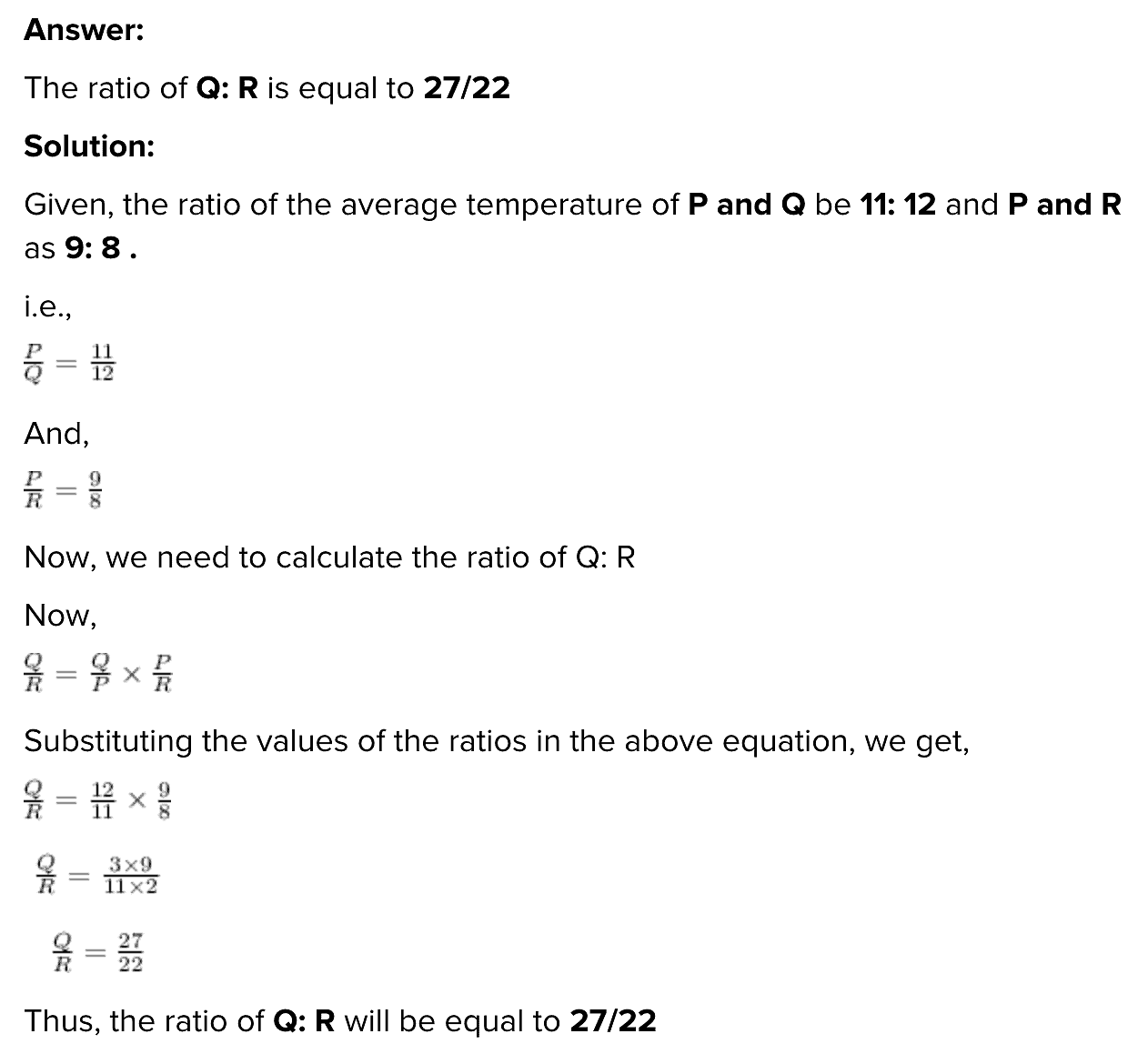Test: Ratio And Proportion, Indices, Logarithms - 1 - Question 19

If x : y = 3 : 4, the value of x2y + xy2 : x3 + y3 is

Detailed Solution for Test: Ratio And Proportion, Indices, Logarithms - 1 - Question 19

From the given data, we have x = (3/4) y or 4x = 3y

(x2y + xy2) :: (x2 + y2) = xy(x + y) / {(x + y)(x2 - xy + y2) = (xy) / (x2 - xy + y2

Substituting x = (3/4)y from the above,

(x2y + xy2) :: (x2 + y2) = (3y2/4) / (9y/ 16 - 3y/ 4 + y2) = 12 / (9 - 12 + 16) = 12/13

Test: Ratio And Proportion, Indices, Logarithms - 1 - Question 20

If p : q is the sub duplicate ratio of p–x2 : q–x2 then x2 is

Detailed Solution for Test: Ratio And Proportion, Indices, Logarithms - 1 - Question 20

√(p-x2) /(q-x2)  = p/q --(1)

[Sub- duplicate ratios of,
a/b= √a/b]

Squaring both sides eq. -(1) ;

(p-x2) /(q-x2) = p2/ q2

q2( p-x2)  = p2(q-x2

q2 p - q2 x2  = p2 q - p2 x2

X2 ( p2-q2) = pq (p-q)

x2 =  pq (p-q) / (p+q) (p-q)

x2 = pq/p+q

Therefore, option (d) none of these is the correct answer.

Test: Ratio And Proportion, Indices, Logarithms - 1 - Question 21

If 2s : 3t is the duplicate ratio of 2s – p : 3t – p then

Detailed Solution for Test: Ratio And Proportion, Indices, Logarithms - 1 - Question 21

If given ratio x : y then it's duplicate

ratio = x^2 : y^2

According to the problem given,

2s / 3t = ( 2s -p)^2 / (3t -p)^2

2s( 3t -p )^2 = 3t( 2s - p )^2

2s( 9t^2 - 6tp + p^2 ) = 3t(4s^2 -4sp+ p^2)

18st^2-12spt+2p^2s= 12s^2t-12spt+3p^2t

18st^2-12s^2t= 3p^2t - 2p^2s

6st(3t -2s) = p^2(3t - 2s)

6st = p^2

Therefore ,

p^2 = 6st

Test: Ratio And Proportion, Indices, Logarithms - 1 - Question 22

If p : q = 2 : 3 and x : y = 4 : 5, then the value of 5px + 3qy : 10px + 4qy is

Detailed Solution for Test: Ratio And Proportion, Indices, Logarithms - 1 - Question 22

The ratio are as follows

p : q = 2 : 3

Let The value of p = 2 a ,

Let The value of q = 3 a

And

x : y = 4 : 5

Let The value of x = 4 b

Let The value of y = 5 b

Now, According to question

Let 5 p x + 3 q y : 10 p x + 4 q y  = m :n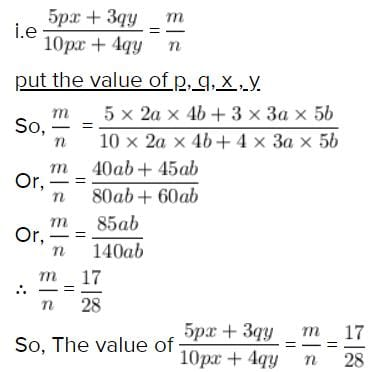Test: Ratio And Proportion, Indices, Logarithms - 1 - Question 23

The number which when subtracted from each of the terms of the ratio 19 : 31 reducing it to 1 : 4 is

Detailed Solution for Test: Ratio And Proportion, Indices, Logarithms - 1 - Question 23

19-x / 31-x =1 / 4
76 - 4x =31 - x
-3x = -45
x=45 / 3
x÷15
so that number is 15.

Test: Ratio And Proportion, Indices, Logarithms - 1 - Question 24

Daily earnings of two persons are in the ratio 4:5 and their daily expenses are in the ratio 7 : 9. If each saves Rs. 50 per day, their daily incomes in Rs. are

Detailed Solution for Test: Ratio And Proportion, Indices, Logarithms - 1 - Question 24

Let the monthly income of one person be 4x and that of the other be 5x

Let the monthly expenses of one person be 7y and that of other be 9y

ATQ

4x-7y=50-(1)-*5=20x-35y=250

5x-9y=50-(2)-*4=20x-36y=200

=y=50

from 1

4x-7*50=50

4x=50+350

4x=400

x=100

Monthly income of one person=4x=4*100=400

Monthly income of the other person=5x=5*100=500

Test: Ratio And Proportion, Indices, Logarithms - 1 - Question 25

The ratio between the speeds of two trains is 7 : 8. If the second train runs 400 Kms. in 5 hours, the speed of the first train is

Detailed Solution for Test: Ratio And Proportion, Indices, Logarithms - 1 - Question 25

Speed of second train = Distance travelled / time = 400 /5 = 80 km/hr
Ratio of speeds = 7 :8 = (7/8) :1
If speed of second train is x, then the speed of first train is 7x/8
7x/8 = 7 x 80/8 = 70
So, speed of first train is 70 km/hr

Test: Ratio And Proportion, Indices, Logarithms - 1 - Question 26

The fourth proportional to 4, 6, 8 is

Detailed Solution for Test: Ratio And Proportion, Indices, Logarithms - 1 - Question 26

For proportions; the product of the internal terms equals the product of the externals.

Therefore,

=> 4 : 8 = 6: x
=> 4x = 48
=> x = 12.

Test: Ratio And Proportion, Indices, Logarithms - 1 - Question 27

The third proportional to 12, 18 is

Detailed Solution for Test: Ratio And Proportion, Indices, Logarithms - 1 - Question 27

Let the third proportion be x

12 : 18 = 18 : x

x = 18*(18/12)

x = 18*(3/2)

x = 54/2

x = 27

Test: Ratio And Proportion, Indices, Logarithms - 1 - Question 28

The mean proportional between 25, 81 is

Detailed Solution for Test: Ratio And Proportion, Indices, Logarithms - 1 - Question 28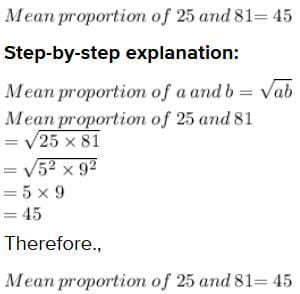Test: Ratio And Proportion, Indices, Logarithms - 1 - Question 29

The number which has the same ratio to 26 that 6 has to 13 is

Detailed Solution for Test: Ratio And Proportion, Indices, Logarithms - 1 - Question 29

The ratio of the number similar to 6∶13 is 12∶26

Given:

One ratio of the number is 6 : 13 which becomes 6/13

To find:

The another ratio of number similar to 6 : 13

Solution:

Let the unknown number be x

Given that another ratio of the number is x: 26 which becomes x/26

Given that the two ratios are similar, equating the two terms, we get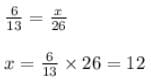Hence the ratio of the number similar to 6∶13 is 12∶26

Test: Ratio And Proportion, Indices, Logarithms - 1 - Question 30

The ratio of the present age of father to that of son is 7:2. After 10 years their ages will be in the ratio of 9:4. The present ages of the father is

Detailed Solution for Test: Ratio And Proportion, Indices, Logarithms - 1 - Question 30

Let father age be X and son age be Y. Then
X/Y = 7/2
2X = 7Y ..... (1)
After ten years ,
(X+10)/(Y+10) = 9/4
4(X+10) = 9(Y+10)
4X + 40 = 9Y + 90
4X = 9Y + 50
2×2X = 9Y + 50

putting the value of 2X in equation 1

2×7Y = 9Y + 50
14Y = 9Y + 50
5Y = 50
Y = 10
Son age is 10 years .

putting the value of Y in equation 1

2X = 7Y
X = 7/2Y
X = 7/2×10
X = 70/2
X = 35
Father age is 35.

Test: Ratio And Proportion, Indices, Logarithms - 1 - Question 31

If four numbers 1/2, 1/3, 1/5, 1/x are proportional then x is

Detailed Solution for Test: Ratio And Proportion, Indices, Logarithms - 1 - Question 31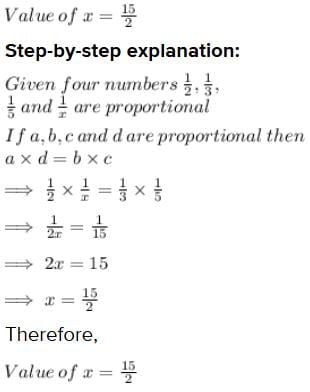Test: Ratio And Proportion, Indices, Logarithms - 1 - Question 32

The mean proportional between 12x2 and 27y2 is

Detailed Solution for Test: Ratio And Proportion, Indices, Logarithms - 1 - Question 32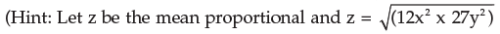Test: Ratio And Proportion, Indices, Logarithms - 1 - Question 33

If A = B/2 = C/5, then A : B : C is

Detailed Solution for Test: Ratio And Proportion, Indices, Logarithms - 1 - Question 33

I think you take all in respect to A and cut it out
∴ a= a
b = 2a
c= 5a
a:b:c = 1:2:5

Test: Ratio And Proportion, Indices, Logarithms - 1 - Question 34

If a/3 = b/4 = c/7, then a+b+c/c is

Detailed Solution for Test: Ratio And Proportion, Indices, Logarithms - 1 - Question 34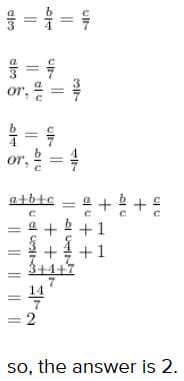Test: Ratio And Proportion, Indices, Logarithms - 1 - Question 35

If p/q = r/s = 2.5/1.5, the value of ps:qr is

Detailed Solution for Test: Ratio And Proportion, Indices, Logarithms - 1 - Question 35

p/q = r/s = 2.5/1.5 = 5/3

Now, ps:qr = ps/qr = 5 x 3/3 x 5 = 1:1

Test: Ratio And Proportion, Indices, Logarithms - 1 - Question 36

If x : y = z : w = 2.5 : 1.5, the value of (x+z)/(y+w) is

Detailed Solution for Test: Ratio And Proportion, Indices, Logarithms - 1 - Question 36

x/y = 5/3

x = 5/3 y

z/w = 5/3

z = 5/3 w

x + z = 5/3 y + 5/3 w

= 5/3( y + w)

(x + z)/(y + w) = 5/3

Test: Ratio And Proportion, Indices, Logarithms - 1 - Question 37

If (5x–3y)/(5y–3x) = 3/4, the value of x : y is

Detailed Solution for Test: Ratio And Proportion, Indices, Logarithms - 1 - Question 37

( 5x - 3y ) / ( 5y - 3x ) = 3/4
Cross multiplying the numbers in the left and right ,
4 (5x - 3y) = 3 (5y - 3x)
Opening the brackets ,
20x - 12y = 15y - 9x
Grouping like terms to one side ,
20x + 9x = 15y + 12y
29x = 27y

→ 29 * x = 27 * y
→ x / y = 27 / 29
→ x:y = 27:29

Test: Ratio And Proportion, Indices, Logarithms - 1 - Question 38

If A : B = 3 : 2 and B : C = 3 : 5, then A:B:C is

Detailed Solution for Test: Ratio And Proportion, Indices, Logarithms - 1 - Question 38

The obtained ratio for A: B: C is 9 : 6 : 10
Given : A: B = 3: 2 and B: C = 3: 5

To compare two ratios, we need to make one of the ratios equal. Here we had B term in both the ratios, so we are making two terms equal by making B equal.

Multiplying A: B i.e. 3: 2 by 3

and B: C i.e. 3: 5 by 2 to bring the value of B at par, we get

A: B = 9: 6 & B: C = 6: 10

Hence, the value of A: B: C is obtained to be 9 : 6 : 10

Test: Ratio And Proportion, Indices, Logarithms - 1 - Question 39

If x/2 = y/3 = z/7, then the value of (2x–5y+4z)/2y is

Detailed Solution for Test: Ratio And Proportion, Indices, Logarithms - 1 - Question 39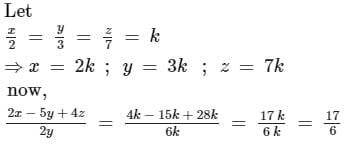Test: Ratio And Proportion, Indices, Logarithms - 1 - Question 40

If x : y = 2 : 3, y : z = 4 : 3 then x : y : z is

Detailed Solution for Test: Ratio And Proportion, Indices, Logarithms - 1 - Question 40

Making y same, we get

x:y = 8 :12 (multiplied by 4)

y:z = 12:9  (multiplied by 3)

x:y:z = 8:12:9

## Business Mathematics and Logical Reasoning & Statistics

131 videos|149 docs|87 tests
 Use Code STAYHOME200 and get INR 200 additional OFF Use Coupon Code
Information about Test: Ratio And Proportion, Indices, Logarithms - 1 Page
In this test you can find the Exam questions for Test: Ratio And Proportion, Indices, Logarithms - 1 solved & explained in the simplest way possible. Besides giving Questions and answers for Test: Ratio And Proportion, Indices, Logarithms - 1, EduRev gives you an ample number of Online tests for practice

## Business Mathematics and Logical Reasoning & Statistics

131 videos|149 docs|87 tests